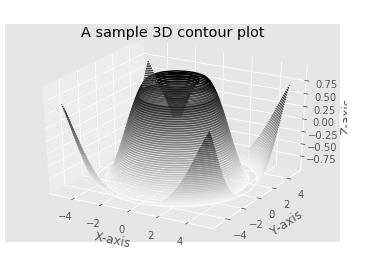# How can Matplotlib be used to create 3 dimensional contour plot using Python?

Matplotlib is a popular Python package that is used for data visualization. Visualizing data is a key step since it helps understand what is going on in the data without actually looking at the numbers and performing complicated computations. It helps in communicating the quantitative insights to the audience effectively.

Matplotlib is used to create 2 dimensional plots with the data. It comes with an object oriented API that helps in embedding the plots in Python applications. Matplotlib can be used with IPython shells, Jupyter notebook, Spyder IDE and so on.

It is written in Python. It is created using Numpy, which is the Numerical Python package in Python.

Python can be installed on Windows using the below command −

pip install matplotlib

The dependencies of Matplotlib are −

Python ( greater than or equal to version 3.4)
NumPy
Setuptools
Pyparsing
Libpng
Pytz
Free type
Six
Cycler
Dateutil

Three dimensional plots are created to view the x−, y− and z−axes of the data points. It can also be used to understand how the gradient descent function works, and to find the optimal values for coefficients for an algorithm.

Let us understand how Matplotlib can be used to create three−dimensional contour plot −

## Example

from mpl_toolkits import mplot3d
import numpy as np
import matplotlib.pyplot as plt
def fun(x, y):
return np.sin(np.sqrt(x ** 2 + y ** 2))

x = np.linspace(−5, 5, 30)
y = np.linspace(−5, 5, 30)

X, Y = np.meshgrid(x, y)
Z = fun (X, Y)

fig = plt.figure()
ax = plt.axes(projection='3d')
ax.contour3D(X, Y, Z, 50, cmap='binary')
ax.set_ylabel("Y−axis")
ax.set_xlabel("X−axis")
ax.set_zlabel("Z−axis")

ax.set_title('A sample 3D contour plot')
plt.show()

## Output## Explanation

• The required packages are imported and its alias is defined for ease of use.

• A function ‘fun’ is created that generates data using the ‘sin’ function using two variables.

• The data values are created using the NumPy library.

• An empty figure is created using the ‘figure’ function.

• The ‘axes’ function is used to create an axis to plot the graph.

• The ‘contour3D’ is used to specify that a 3−dimensional contour plot is being visualized with the data that has been created.

• The set_xlabel, set_ylabel, ‘z_label’ and set_title functions are used to provide labels for ‘X’ axis, ‘Y’ axis, Z-axis and title.

• It is shown on the console using the ‘show’ function.